# Homework

Mother and Kate together weigh 24.5 kg more than the father. Father weighs 7.6 kg more than mother. Father weight 44.8 kg more than Kate. Determine weights of father mother and Kate.

k =  32.1
m =  69.3
o =  76.9

### Step-by-step explanation:

m+k=o+24.5
o = m + 7.6
o = k + 44.8

k+m-o = 24.5
m-o = -7.6
k-o = -44.8

k = 321/10 = 32.1
m = 693/10 = 69.3
o = 769/10 = 76.9

Our linear equations calculator calculates it.Did you find an error or inaccuracy? Feel free to write us. Thank you!Tips to related online calculators
Do you have a linear equation or system of equations and looking for its solution? Or do you have a quadratic equation?

## Related math problems and questions:

• Father and sonFather and son weigh together 108kg. The father weighs 2.6 times more than his son. How much does weight my father and son count?
• Can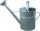Watering can full of water weighs 10 kg. Half-full can weighs 5.5 kg. How much weigh can?
• WeightsMarry and John together weighing 49 kg. Their weights are in ratio 1:6. Determine their weights.
• Children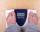Margaret and Zdena weigh the same and Petra 3 kg more. Together weigh 156 kg. How much do they weigh?
• Barrel 4Barrel of water weighs 63 kg. After off 75% water, the weight of the barrel with water is 21 kg. How many kg weigh empty barrel and how many kgs water in it?
• Two melons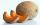There are 2 melons in the box. Together they weigh 11kg. The weight of green is 3 kg less than three times the weight of yellow. What is the weight of a green melon?
• Watering can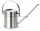A watering can full of water weighs 11 kg, the weight of water is 10 kg greater than the weight of an empty watering can, how much is the weight of the watering can?
• Reservoir + waterThe reservoir filled with water weighs 12 kg and after pouring off three-quarters of the water weights 3 kg. Calculate the weight and volume of the reservoir.
• BucketThe bucket half filled with water weighs 5.55 kg, the full bucket weighs 9.85 kg. How much does the bucket weigh?
• Balance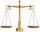The rod are 1.9 m long hanging weights 4 kg and 1 kg on ends. Where are centre of rod (distance from weight 4 kg) to be in balance?
• Bags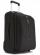The first bag has a weight of 18 kg, the second bag is a one-third lighter than the first and the third bag has three times less the weight of all three bags together. Determine their weights.
• Barrel 3Barrel with water weights 118 kg. When we get off 75% of water, it weights 35 kg. How many kg has an empty barrel?
• Tess boughtTess bought 6 dressed chickens. Their weights are tagged as follows: 1.4 kg, 1.9 kg, 2.05 kg, 0.94 kg, 1.1 kg and 1.45 kg. What is the total weight of the six chickens
• Container with water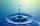The weight of a container with water is 2.48 kg. If the cast 75% water, the container with water has a weight of 0.98 kg. Determine the weight of the empty container. How much water was originally in the container?
• The water barrel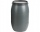The water barrel weighs 122 kg. If we pour 75% of the water out of it, it will weigh 35 kg. What is the weight of the barrel?
• Trio weight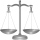Adelka, Barunka, and Cecilka are weight in pairs. Adelka with Barunka weighs 98 kg, Barunka with Cecilka 90 kg and Adelka with Cecilka 92 kg. How much does each of them weigh?
• The devils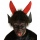The devils weighed in hell with Dorota. They found that Dorota and the two devils weigh 250 kg together, and Dorota and the four devils weigh 426 kg. All the devils weigh the same. How Much Does Dorota Weigh?